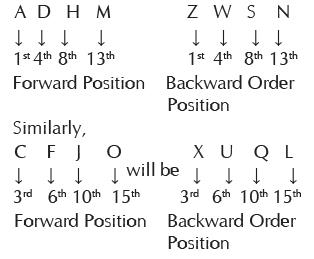Courses

# Olympiad Test : Analogy - 2

## 20 Questions MCQ Test | Olympiad Test : Analogy - 2

Description
This mock test of Olympiad Test : Analogy - 2 for Class 5 helps you for every Class 5 entrance exam. This contains 20 Multiple Choice Questions for Class 5 Olympiad Test : Analogy - 2 (mcq) to study with solutions a complete question bank. The solved questions answers in this Olympiad Test : Analogy - 2 quiz give you a good mix of easy questions and tough questions. Class 5 students definitely take this Olympiad Test : Analogy - 2 exercise for a better result in the exam. You can find other Olympiad Test : Analogy - 2 extra questions, long questions & short questions for Class 5 on EduRev as well by searching above.
QUESTION: 1

### Melt : Liquid :: Freeze : ?

Solution:

Melting of ice changes in liquid  and Freezing of liquid changes in ice.

QUESTION: 2

### Thread : Cloth:: Wire : ?

Solution:

Cloth is made of Threads in the same way Mesh is mode of wire.

QUESTION: 3

### Scissors : Cloth :: ?

Solution:

Scissors is used to cut Cloth in the same way Axe is used to cut wood.

QUESTION: 4

Chisel : Sculptor :: Harrow : ?

Solution:

Chisel is used by Sculptor in the same way Harrow is used by Gardener.

QUESTION: 5

Moon : Satellite :: Earth : ?

Solution:

Moon is a Satellite in the same way Earth is a Planet.

QUESTION: 6

Umpire : Game :: ?

Solution:

In a game Umpire takes final decision, similarly in a debate Moderator takes final decision.

QUESTION: 7

Select the related word/ number from the given alternatives.

CFIL : ORUX :: DGJM : ?

Solution: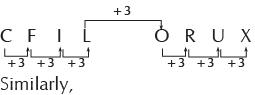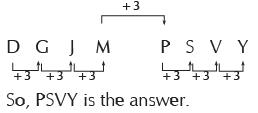QUESTION: 8

Select the related word/ number from the given alternatives.

BEHK : YVSP : : DGJM : ?

Solution:

In this question, Right hand side alphabets have corresponding position in reverse order.

QUESTION: 9

Select the related word/ number from the given alternatives.

AZCX : BYDW :: HQ JO : ?

Solution: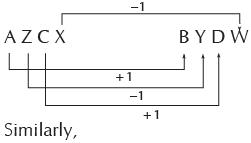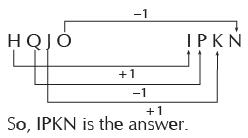QUESTION: 10

Select the related word/ number from the given alternatives.

QIOK : MMKO:: YAWC : ?

Solution: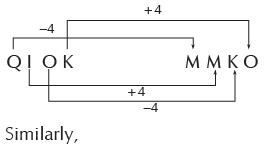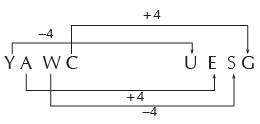QUESTION: 11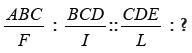Solution: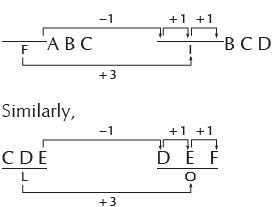QUESTION: 12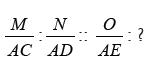Solution:
QUESTION: 13

Select the related word/ number from the given alternatives.

ADBC : EHFG : : ILJK : ?

Solution: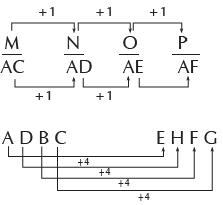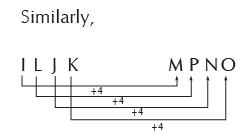QUESTION: 14

Select the related word/ number from the given alternatives.

FGHI : OPQR :: BCDE : ?

Solution: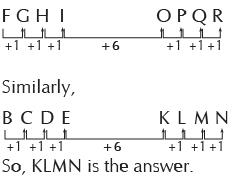QUESTION: 15

Select the related word/ number from the given alternatives.

PNLJ : IGEC :: VTRP : ?

Solution: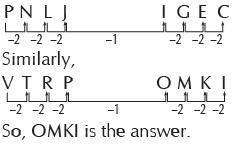QUESTION: 16

Select the related word/ number from the given alternatives.

ABE : 8 :: KLO : ?

Solution:

ABE : 8
The positions of A, B and E in English alphabet are1,2 and 5 respectively.
By adding these, 1+2+5 = 8
Similarly, the positions of K,L and O
in English alphabet are 11, 12 and 15 respectively.

QUESTION: 17

Select the related word/ number from the given alternatives.

EGIK : FILO :: FHJL : ?

Solution: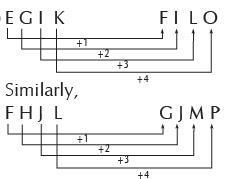QUESTION: 18

Select the related word/ number from the given alternatives.

DRIVE : EIDRV :: BEGUM : ?

Solution: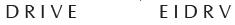If we give number to all alphabets, we get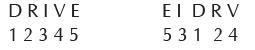similarly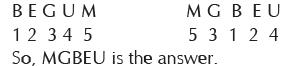QUESTION: 19

Select the related word/ number from the given alternatives.

LGH : KKI :: CIA : ?

Solution: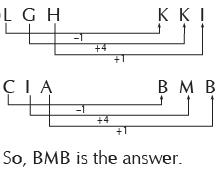QUESTION: 20

Select the related word/ number from the given alternatives.

ADHM : ZWSN :: CFJO : ?

Solution: